##### The Japanese Bonsai specialist
Direct order Contact Help / Services Newsletter# Pinus pentaphylla 28070221

Pinus pentaphylla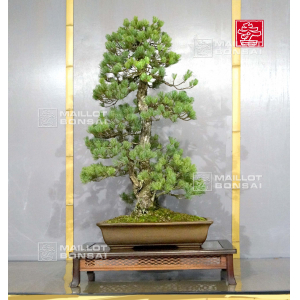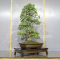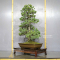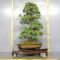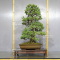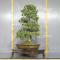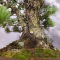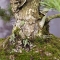ref. : 99063.840,00

voluminous/heavy item extra shipping of 12,00

Available quantity : 1Order

###### Description

Height of the tree without its pot : 880 mm. Width of the branches : 520 mm. Japanese artisanal quality non-enamelled pottery of : 485 x 340 x 110 mm. Kabudachi style. Pine with a graceful silhouette, a large trunk perfectly conical and  proportional smaller trunks without any wire mark or cutting wound. The diameter of the trunks amounts 80 x 150 mm.

Pinus species from seeding, selected for their small-sized needles, their aspect and their green-blue color. Composed of a formed bark because this subject is aged of more than 50/60 years old. From master Tomoya Nishikawa’s nurseries. Exceptional subject, really uncommon! Admire the beauty of its 4 trunks perfectly conical, with their formed bark and their nebari which makes this bonsai unique.

Recently formed in february 2018 by master Tomoya Nishikawa in order to refine its beauty.

It’s really rare to find, even in Japan, 5-needles-pines with so much grace, prompting to the spectator a real emotion and even more : in exceptional piece of this type.

These pictures were taken in september 2022.

#their 5.5 #trunks 3.6 #formed 3.6 #bonsai 3.6 #pinus 3.5 #this 3.4 #with 3.4 #masterpieces 3.2 #pentaphylla 3.1 #exceptional 3.1

Formule
(( ROUND((CHAR_LENGTH(b.article_nom)-CHAR_LENGTH(REPLACE(b.article_nom, 'their', '')))/LENGTH('their')) + ROUND((CHAR_LENGTH(b.article_description)-CHAR_LENGTH(REPLACE(b.article_description, 'their', '')))/LENGTH('their')) ) * 5.5) + (( ROUND((CHAR_LENGTH(b.article_nom)-CHAR_LENGTH(REPLACE(b.article_nom, 'trunks', '')))/LENGTH('trunks')) + ROUND((CHAR_LENGTH(b.article_description)-CHAR_LENGTH(REPLACE(b.article_description, 'trunks', '')))/LENGTH('trunks')) ) * 3.6) + (( ROUND((CHAR_LENGTH(b.article_nom)-CHAR_LENGTH(REPLACE(b.article_nom, 'formed', '')))/LENGTH('formed')) + ROUND((CHAR_LENGTH(b.article_description)-CHAR_LENGTH(REPLACE(b.article_description, 'formed', '')))/LENGTH('formed')) ) * 3.6) + (( ROUND((CHAR_LENGTH(b.article_nom)-CHAR_LENGTH(REPLACE(b.article_nom, 'pinus', '')))/LENGTH('pinus')) + ROUND((CHAR_LENGTH(b.article_description)-CHAR_LENGTH(REPLACE(b.article_description, 'pinus', '')))/LENGTH('pinus')) ) * 3.5) + (( ROUND((CHAR_LENGTH(b.article_nom)-CHAR_LENGTH(REPLACE(b.article_nom, 'with', '')))/LENGTH('with')) + ROUND((CHAR_LENGTH(b.article_description)-CHAR_LENGTH(REPLACE(b.article_description, 'with', '')))/LENGTH('with')) ) * 3.4) + (( ROUND((CHAR_LENGTH(b.article_nom)-CHAR_LENGTH(REPLACE(b.article_nom, 'this', '')))/LENGTH('this')) + ROUND((CHAR_LENGTH(b.article_description)-CHAR_LENGTH(REPLACE(b.article_description, 'this', '')))/LENGTH('this')) ) * 3.4) + (( ROUND((CHAR_LENGTH(b.article_nom)-CHAR_LENGTH(REPLACE(b.article_nom, 'pentaphylla', '')))/LENGTH('pentaphylla')) + ROUND((CHAR_LENGTH(b.article_description)-CHAR_LENGTH(REPLACE(b.article_description, 'pentaphylla', '')))/LENGTH('pentaphylla')) ) * 3.1) + (( ROUND((CHAR_LENGTH(b.article_nom)-CHAR_LENGTH(REPLACE(b.article_nom, 'exceptional', '')))/LENGTH('exceptional')) + ROUND((CHAR_LENGTH(b.article_description)-CHAR_LENGTH(REPLACE(b.article_description, 'exceptional', '')))/LENGTH('exceptional')) ) * 3.1) + (( ROUND((CHAR_LENGTH(b.article_nom)-CHAR_LENGTH(REPLACE(b.article_nom, 'perfectly', '')))/LENGTH('perfectly')) + ROUND((CHAR_LENGTH(b.article_description)-CHAR_LENGTH(REPLACE(b.article_description, 'perfectly', '')))/LENGTH('perfectly')) ) * 2.9) + (( ROUND((CHAR_LENGTH(b.article_nom)-CHAR_LENGTH(REPLACE(b.article_nom, 'nishikawa', '')))/LENGTH('nishikawa')) + ROUND((CHAR_LENGTH(b.article_description)-CHAR_LENGTH(REPLACE(b.article_description, 'nishikawa', '')))/LENGTH('nishikawa')) ) * 2.9)

## Secure payment## Delivery

Our logistic partners :04 74 55 23 48
Pépinière MAILLOT-BONSAÏ
Le Bois Frazy
01990 RELEVANT - FRANCE
on appointment